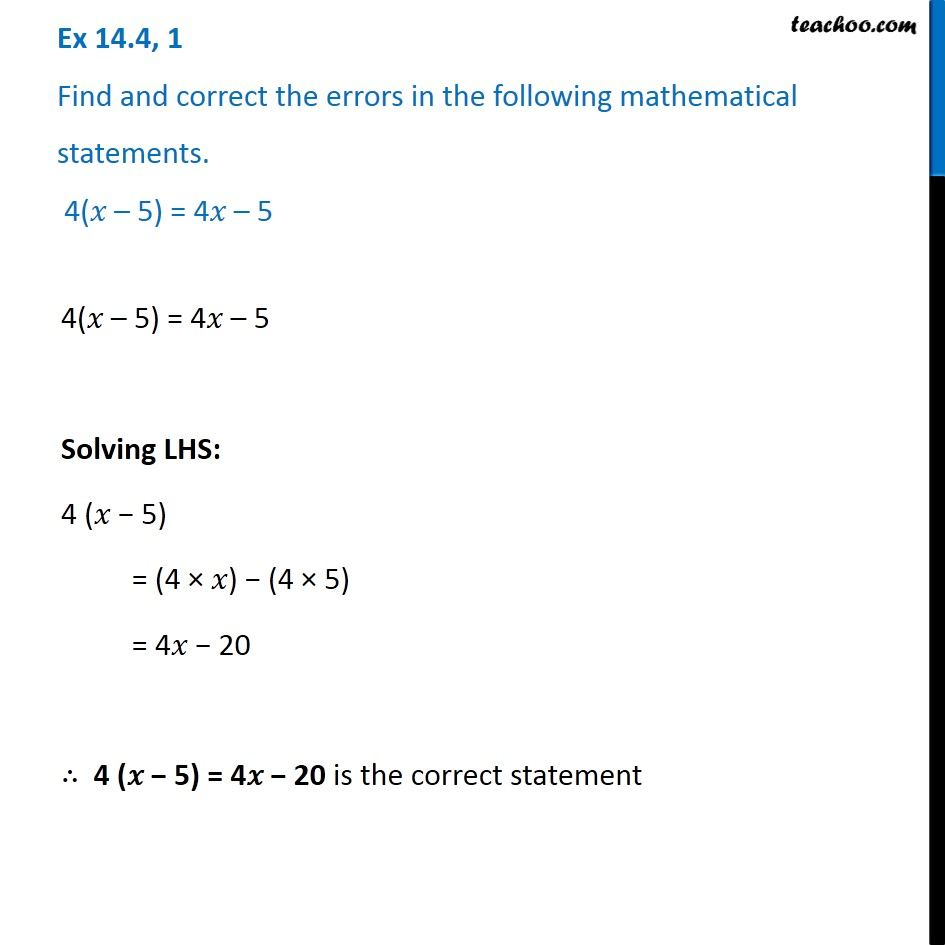Identify and correct errors in the sentences

Chapter 12 Class 8 Factorisation
Serial order wiseLearn in your speed, with individual attention - Teachoo Maths 1-on-1 Class

### Transcript

Question 1 Find and correct the errors in the following mathematical statements. 4(𝑥 – 5) = 4𝑥 – 5 4(𝑥 – 5) = 4𝑥 – 5 Solving LHS: 4 (𝑥 − 5) = (4 × 𝑥) − (4 × 5) = 4𝑥 − 20 ∴ 4 (𝑥 − 5) = 4𝑥 − 20 is the correct statement